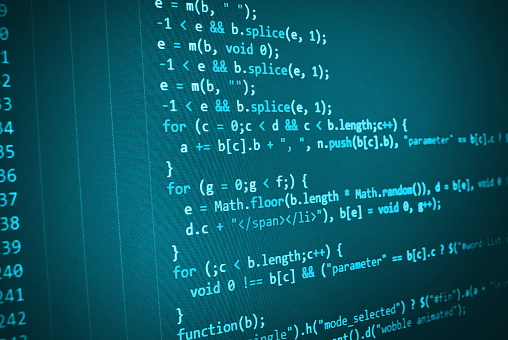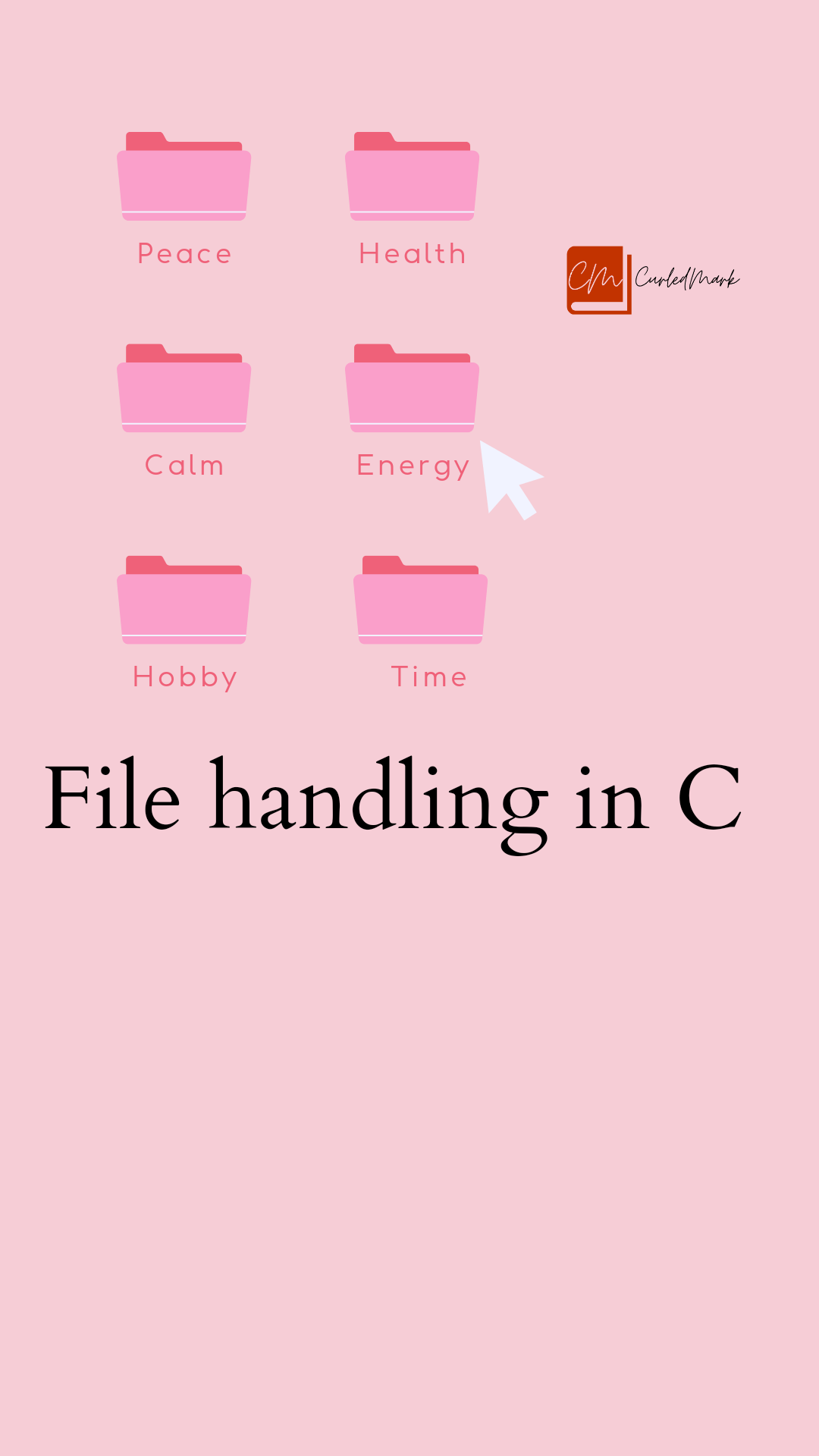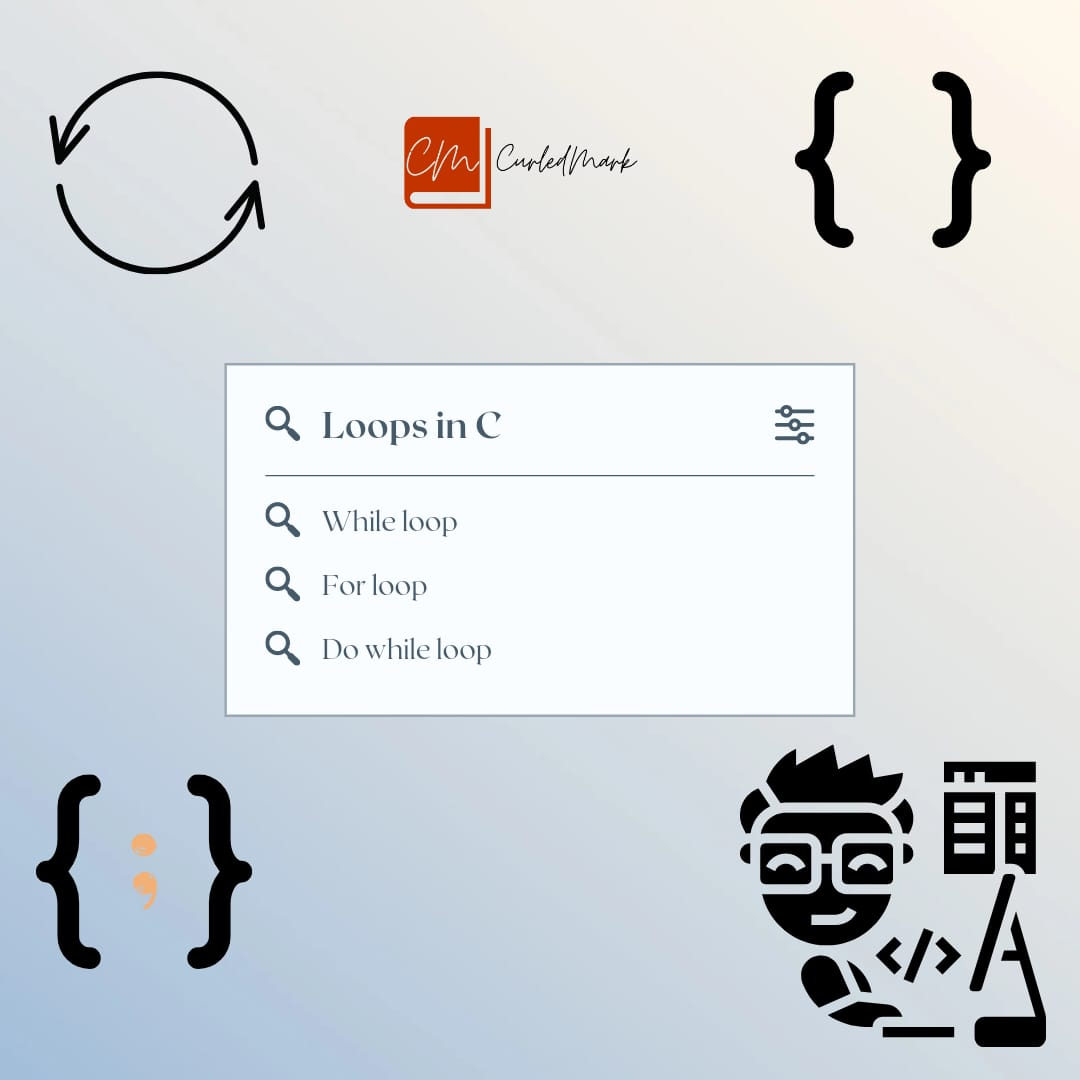January 31, 2023Software developer programming code on computer. Abstract computer script source code.

# Operators and accepting input from Keyboard in C Programming

## C Programming

This article contains the second part of the C programming basics. It explains all the important operators to be used in the C Programming language.

In the second part of it, there is an explanation of how one can accept input from integers.

## What is this symbol(\n) in C Programming?

\n is a new line character. It directs the cursor to the next line. You can see the result of using \n in the output below.

#include<stdio.h>

int main()

{

int x;

int y;

int z;

x=10;

y=20;

z=x+y;

Printf(“The value of x is %d\n”,x);

Printf(“The value of y is %d\n”,y);

Printf(“The Total is %d”,z);

return 0;

}

Output:

The value of x is 10

The value of y is 20

The Total is z is 30

## Operators in C programming

There are different types of operators that we use while writing a program. So we should know about all the operators’ functionality.

Assignment Operators

Equals (=): Equals operator is used to assigning a value to a variable.

x=10

Arithmetic Operators

• Subtraction(-)
• Multiplication(*)
• Division(/)
• Modulo(%)

The modulo operator outputs the remainder part of the division operation.

10%3=1, 10%2=0,10%15=10

• ++

x++ => x=x+1

x– => x=x-1

• +=

x+= =>  x+=10 => x=x+10

• -+

x-+ => x-=5 => x=x-5

• *=

x*= => x*=2 => x=x*2

• /=

x/= => x/=2 => x=x/2

• %=

x%= => x%=2 => x=x%2

Relational Operators

Less than(<)

Greater than(>)

Less than equal to(<=)

Greater than equal to(>=)

Equality Operators:

==

!=

Logical Operators

• And Operator(&&)

T T T

T F F

F T F

F F F

• OR Operator(||)

T T T

T F T

F T T

F F F

From the previous article, we have known that OS allocates memory in RAM where the variable is stored in an address.

Fundamentals of C Programming in simple way

Suppose the address where x is stored is 175. So 175 is the address for x. The address is stored in &x, this is how the address storing is denoted.

int x;

x=20;

&x=175;

Therefore the value of x is 20 and the address of x is 175.

## How to accept input from Keyboard in C Programming?

What is actually accepting input from the keyboard? It is like we enter the inputs in the output screen of the program and it takes the input after we type it in keyboard. Then it produces the output.

There is another predefined function as Printf, it is called Scanf.

It is a predefined function that can put the application in wait mode to accept input from the keyboard.

#include<stdio.h>

int main()

{

int x,y,z;

Printf(“Enter a number:”);

Scanf(“%d”,&x);

Printf(“Enter another number:”);

Scanf(“%d”,&y);

z=x+y;

Printf(“Total is %d”,z);

return 0;

}

Output Screen:

Enter a number: 10 #The Programmer enters the number after “Enter a number is prompted on the screen”

Enter another number: 20 #Programmer enters the number after “Enter another number is prompted on the screen”

Total is 30 #Then this output is shown

## Conclusion

This article contained all the operators and explained how you can accept the inputs from the keyboard. We are into fundamentals now. Slowly and gradually we will learn all the concepts of C programming. Happy Learning!

The Starless Sea Book Summary by Erin Morgenstern

How can you be more confident? || Confidence

3 PILLARS OF CONFIDENCE || SELF ESTEEM

#### You may have missed#### RIYA SHUKLA, DIVYANSH DWVEDI & CHITRANSH RAAJ TO SHARE SCREEN IN FILM PULLU